# rolerman-Asemic

A Handwritten Letter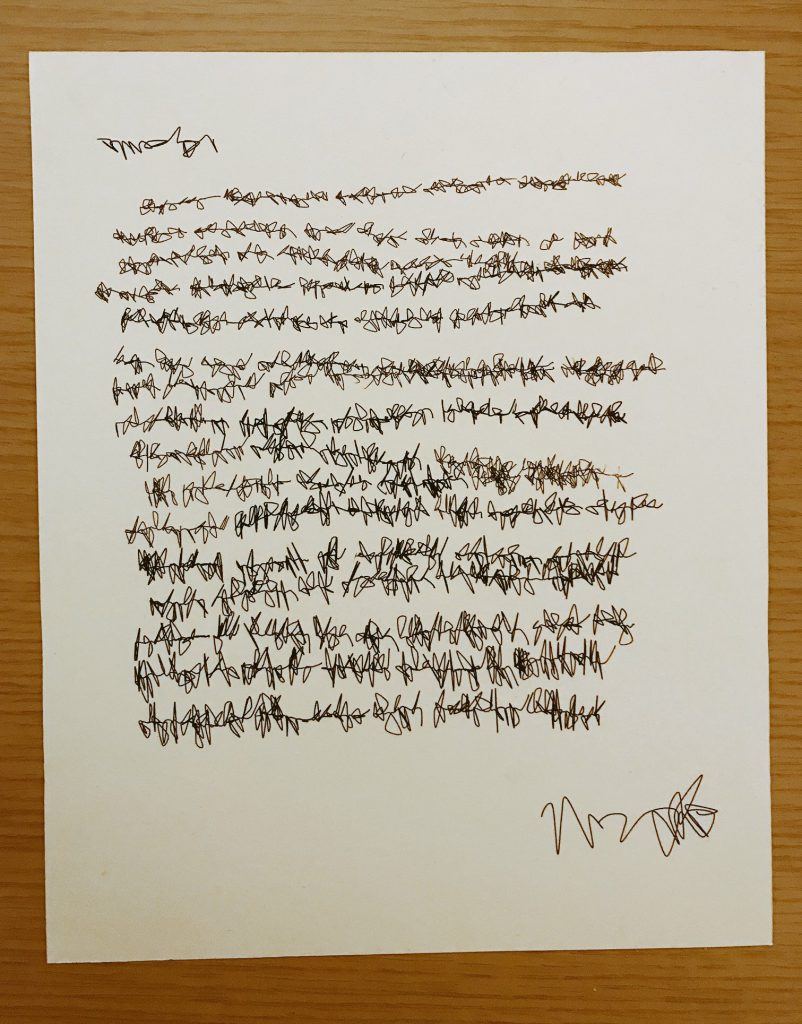I started off thinking about generating lines with some irregularity to them. The first thing I tried was just a series of line segments wobbling up and down.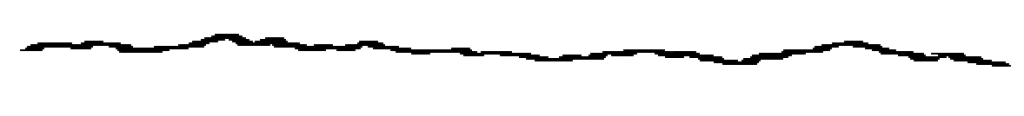Then, I introduced the concept of “words”, creating spaces between the segments.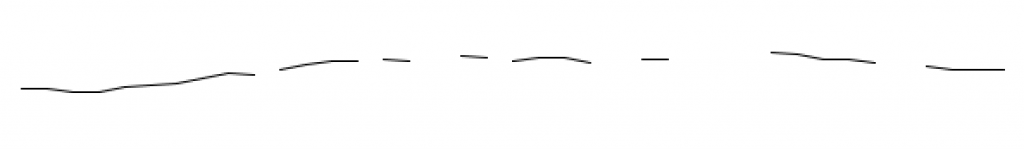I played around with the vertical irregularity of it, so that randomness can add up towards the end of the line.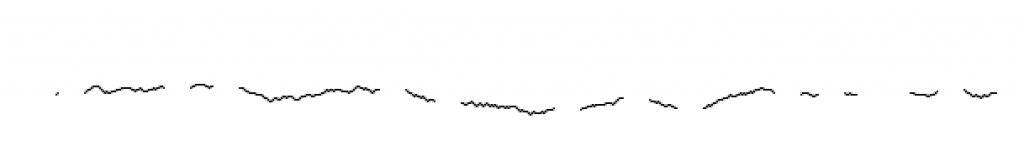I got bored of straight lines to connect the dots, and introduced curves. From here, I was really able to play around with character styles. By varying random parameters such as the “curviness” in the x and y directions, many different sorts of shapes and writings emerge.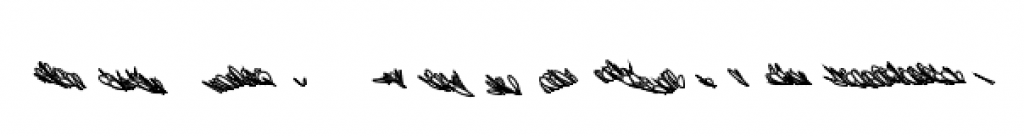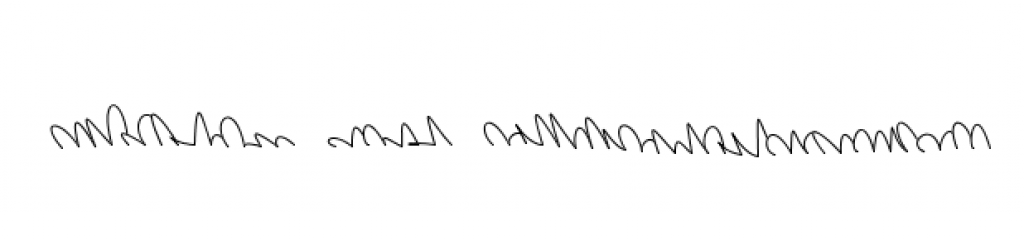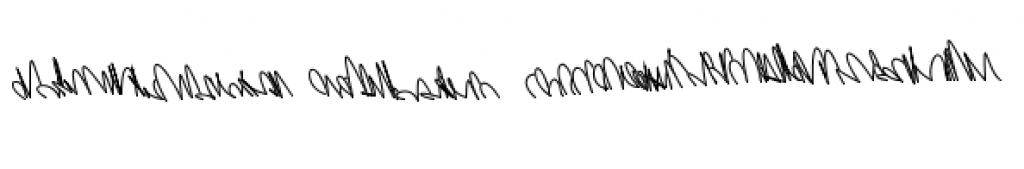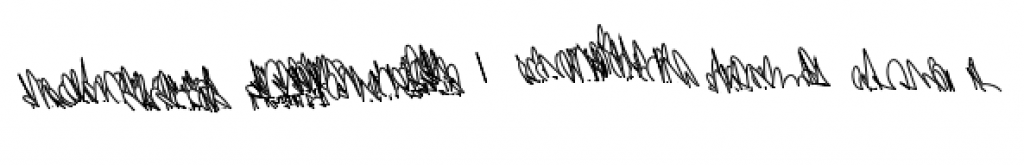From here, I introduced multiple lines of text.I played with varying the parameters of the text as the program was executed.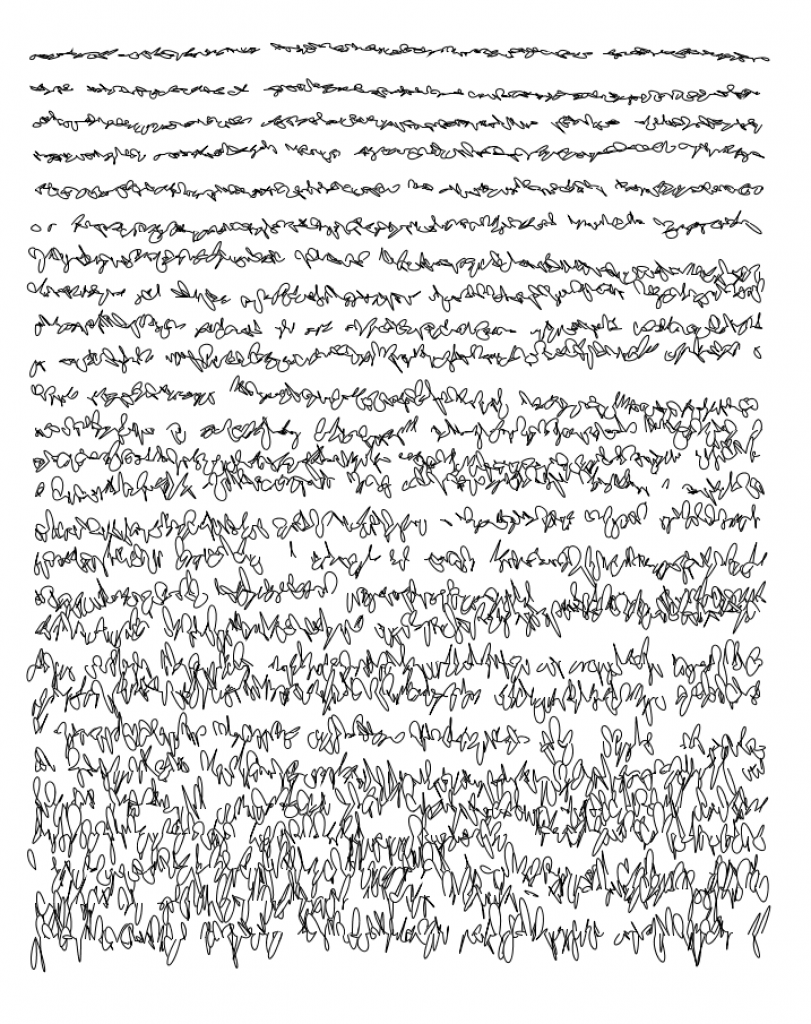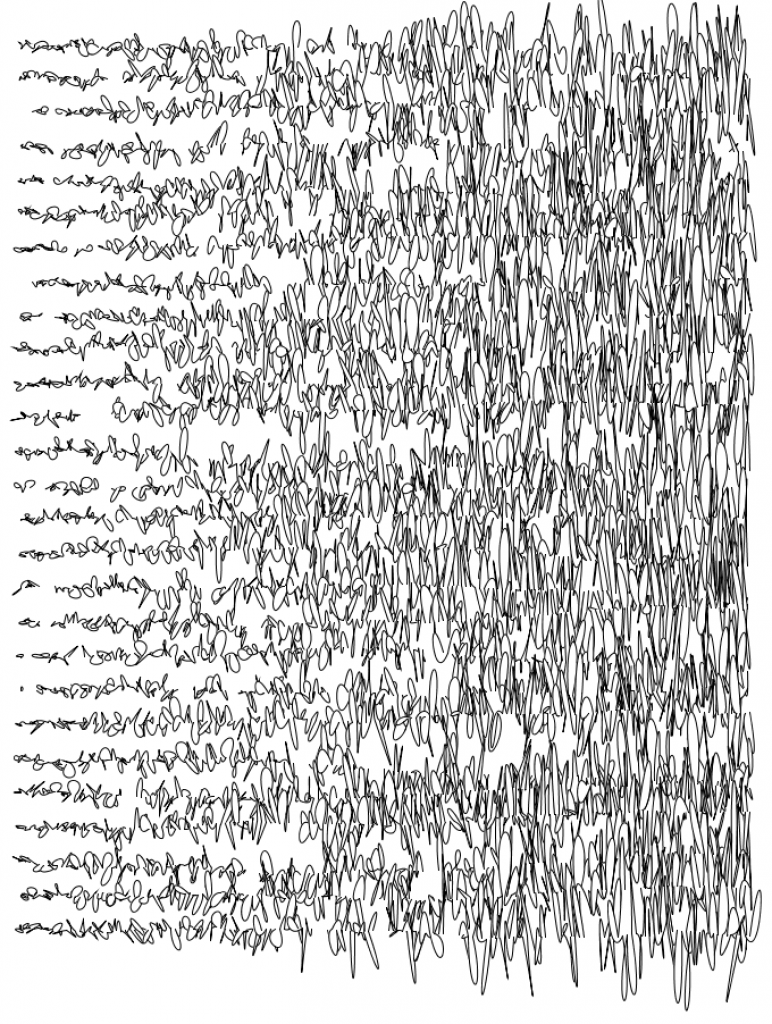Ultimately, this resembles a page of messy handwriting. I wanted to contextualize the writing in the form of something that is often personal and scribbly – a letter. By varying the parameters of the text, I created a “Dear” and “Sincerely” section of the letter, including a signature at the bottom. I also introduced indents at various points to resemble paragraphs. It struck me how much changing the different parameters changed the personality and emotion of the letter.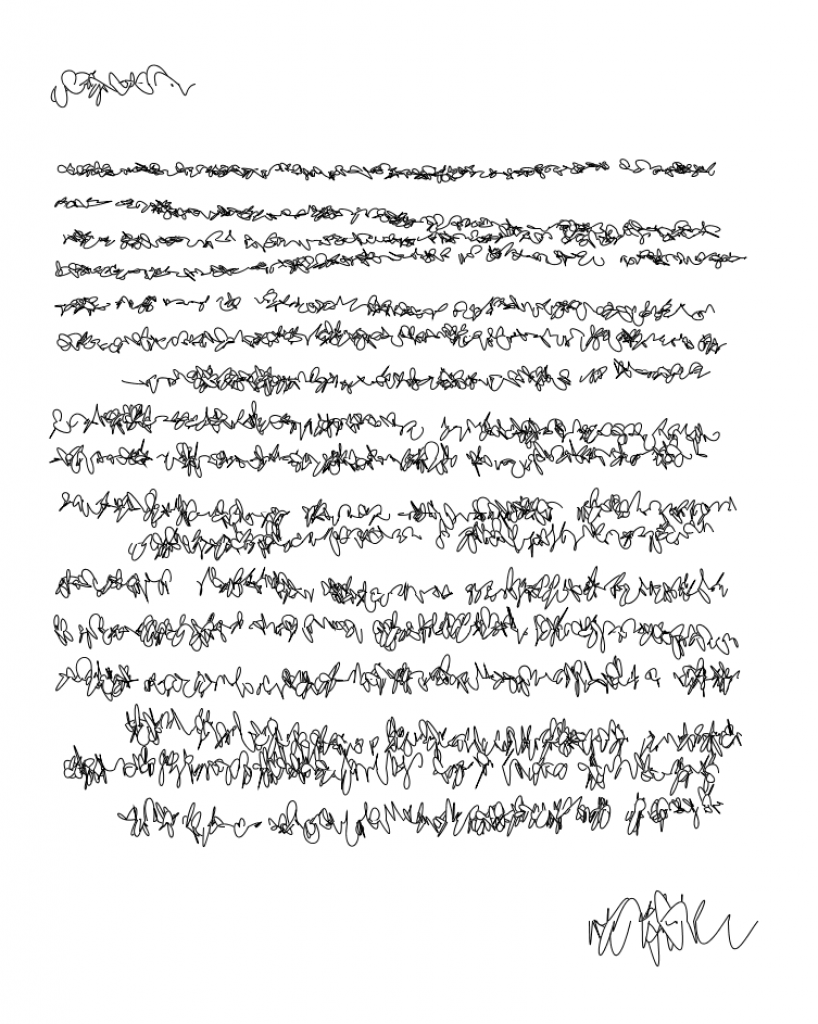Plotting

I generated many letters, and selected the following one to plot. I like the way the lines run into each other at the end, and the signature looks convincingly like real handwriting.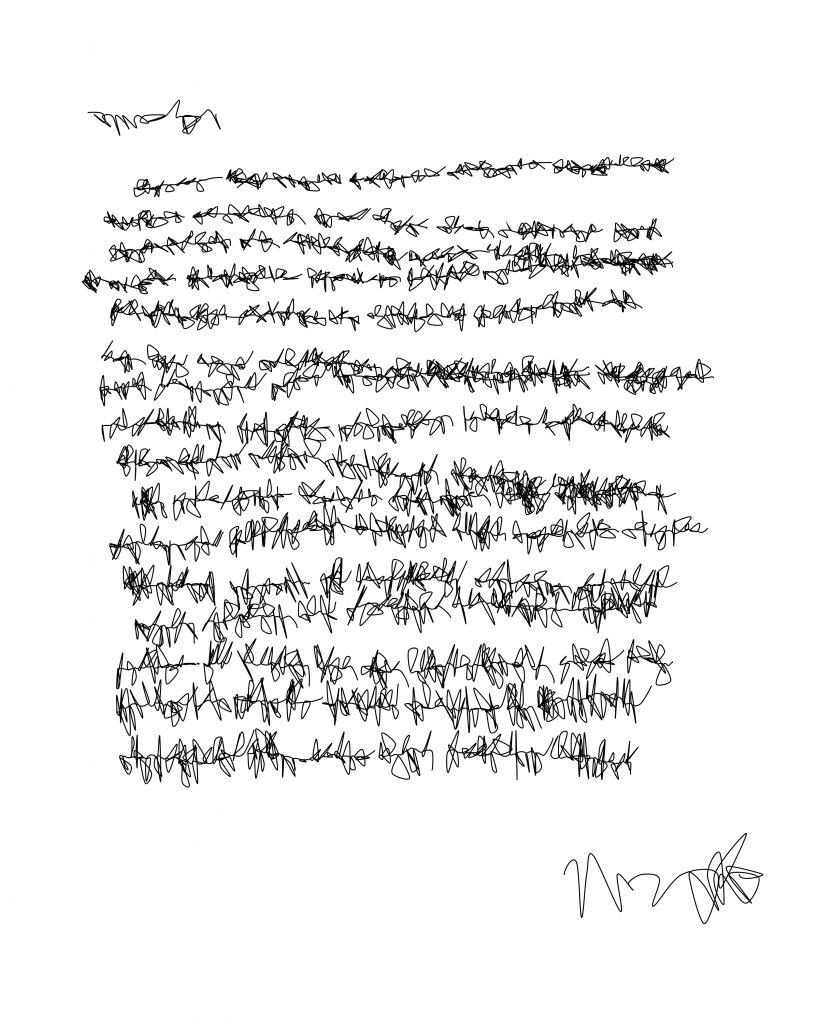The plotter left some holes in my letter where the pen stopped writing. In order to fix this, I ran the plotter multiple times with different portions of the letter file deleted, until all of the text was filled in.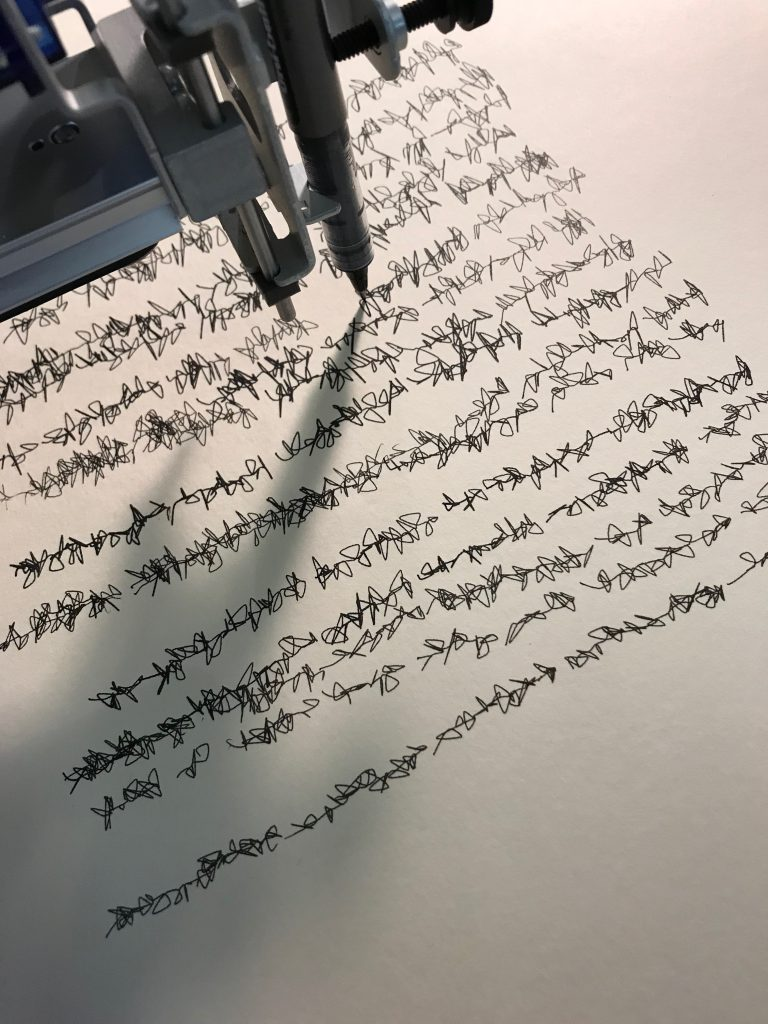Code

Parameters I wound up playing with:

• X character loopiness
• Y character loopiness
• width of each word
• width of each character
• irregularity of the line of text
• where the line of text ends
• where the line of text begins
• spacing between lines of text
```void setup() { size(800, 1000); background(255); noFill(); drawText(); }   int margin = 80; int curveAmountY = 10;   int drawWord(int x, int y, int xmax) { beginShape(); int incMax = 10; float yVar = 3; int currX = x; int currY = y; int nextX = currX + int(random(incMax)); int nextY = currY + int(random(-yVar, yVar)); while (currX < xmax) { int curveAmountX = 12; curveVertex(currX, currY); curveVertex(nextX + int(random(curveAmountX)), nextY + int(random(-curveAmountY, curveAmountY))); curveVertex(currX + int(random(curveAmountX)), currY + int(random(-curveAmountY, curveAmountY))); curveVertex(nextX, nextY); currX = nextX; nextX += int(random(incMax)); currY = nextY; nextY += int(random(-yVar, yVar)); } endShape(); return currY; }   void drawLineOfText(int vertPos) { int currX = margin + int(random(50)); int currY = vertPos; int maxWordWidth = 100; int minWordWidth = 20; int spaceWidth = 10;   int indentWidth = 60; if (random(100) < 10) { // draw an indent currX += indentWidth; }   while (currX < width - margin - maxWordWidth) { int wordWidth = int(random(maxWordWidth)) + minWordWidth; currY = drawWord(currX, currY, currX + wordWidth); currX = currX + wordWidth + spaceWidth; } curveAmountY += 2; }   void drawDear(int vertPos) { beginShape(); int incMax = 20; float yVar = 3; int currX = margin; int currY = vertPos; int nextX = currX + int(random(incMax)); int nextY = currY + int(random(-yVar, yVar)); while (currX < 200) { int curveAmountX = 20; int curveAmountY = 20; curveVertex(currX + int(random(curveAmountX)), currY + int(random(curveAmountY))); curveVertex(nextX, nextY); curveVertex(nextX + int(random(curveAmountX)), nextY + int(random(-curveAmountY, curveAmountY))); currX = nextX; nextX += int(random(incMax)); currY = nextY; nextY += int(random(-yVar, yVar)); } endShape(); }     void drawSignature(int vertPos) { int incMax = 40; float yVar = 0.5; int currX = width - margin - 200; int currY = vertPos; int nextX = currX + int(random(incMax)); curveAmountY += int(random(20)); int nextY = vertPos + int(random(-yVar, yVar));   beginShape(); while (currX < width - margin ) { int curveAmountX = 40; int curveAmountY = 50; curveVertex(currX + int(random(curveAmountX)), currY + int(random(curveAmountY))); //curveVertex(currX, currY); curveVertex(nextX, nextY); curveVertex(nextX + int(random(curveAmountX)), nextY + int(random(-curveAmountY, curveAmountY))); currX = nextX; nextX += int(random(incMax)); currY = nextY; nextY += int(random(-yVar, yVar)); } endShape(); }   void drawText() { int heightSpacing = 30; int y = margin + heightSpacing;   drawDear(y); y += heightSpacing * 2.5;   while (y < height - margin - heightSpacing * 3) { drawLineOfText(y); y += heightSpacing; heightSpacing += 1; } y += heightSpacing*1.5;   drawSignature(y); exit(); }```

Inspiration

I drew a lot of inspiration from this really lovely piece by Vera Molnar called “Letters From my Mother” done in 1988. She writes beautifully about the chaos and urgency that can be found in handwriting. The emotion of a letter is something I thought about a lot during this project.“My mother had a wonderful hand-writing. There was something gothic in it (it was the style of writing of all well-educated ladies in the Habsburg monarchy in the early XXth century) but also something hysteric. The beginning of every line, on the left side, was always regular, severe, gothic and at the end of each line it become [sic] more nervous, restless, almost hysteric. As the years passed, the letters in their totality, become [sic] more and more chaotic, the gothic aspect disappeared step by step and only the disorder remained. Every week she wrote me a letter, it was a basic and important event in my visual environment. They were more and more difficult to decipher, but it was so nice to look at them… then, there were no more letters…So I went on to write letters of hers to myself, on the computer of course, simulating the transition between gothic and hysteric.” – Vera Molnar’s website